# Scalable Permutations!The Heart of Artificial Intelligence

Recently, I received many questions regarding exhaustive combinations using linear data structures such as arrays or character strings. In particular questions like:

"Does an algorithm exist that only uses iteration (loops) to compute all possible combinations of N distinct items?" (Such that no recursion is used.)

"How do I produce all possible combinations of a character string without repeating a sequence?"

"Is there a function or class that returns just two index values (j, i) such that SWAP(a[j], a[i]) always produces a unique combination within an array a[]?" ~ Dr. Elaine R. Milito
(For the Solution Review the Meta-Permutation Class)

"Does a quick, simple, and very efficient permutation algorithm exist that exhausts all possible paths in the Traveling Salesman Problem (TSP) for any linear data structure?"

"Is there a permutation algorithm (a computer program) that completely avoids sorting and does not examine my target array?"

"If I forgot my password, can I write a computer program to decode it?"
(Note: All passwords are countable utilizing the entire character set. A much smaller subset is a countable permutation utilizing the Base-N-Odometer model.)

All of the questions presented above have one core solution: Define a procedure for generating all possible ways of rearranging N distinct items. To answer these questions I had to dig deep into my archive bag for a personal computer project I developed in the spring of 1991. Although avoiding recursion improves speed and minimizes memory usage, time is still the enemy here.

My intention was to gain a familiarity of a linear array permutation without the use of recursion. In particular, several "Example" functions independently demonstrate various iterative brute-force procedures to compute all unique combinations of any linear array type or of any character string.

The overall goal is to swap only two elements in the target array such that a unique combination is produced. The five independent C++ example programs presented here are based upon the following guidelines: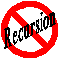Absolutely NO recursion shall be used. It's a well-known fact that iterative algorithms (using loops) are much more efficient than recursive algorithms that do the same thing. A true recursive function is slower and will consume more system resources (especially memory) than its iterative counterpart. Clearly all recursive algorithms have manageable iterative algorithm counterparts, but to confidently imply the opposite that all efficient iterative algorithms have recursive algorithm counterparts is undoubtedly a false statement. In this regard, the vast universe of diverse iterative algorithms will always envelop and completely overwhelm the infinitesimal recursive algorithm universe. (Recursive algorithms are only small seeds from which certain iterative algorithms stem: Try converting MetaPerm to a truly equivalent recursive algorithm without loosing the original intent.)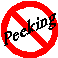The linear permutable target can NOT be examined. Unlike lexicographic (alphabetic) ordering algorithms and iterative heuristic solution techniques which suffer from data dependency; the applications presented here use a single nested loop to create only two indexes (j, i) without scanning the target array or the target character string. To insure a unique combination, a swap(a[j], a[i]) is performed on the target before retrieving the next two new index values. The process completes when an index value exceeds a boundary of the target object list.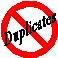Each combination produced will not be repeated. Consecutive swaps on the target array will insure a unique permutation set (where one swap produces a new ordered list). Review the QuickPerm Reversal Algorithm to omit palindromes found within the linear permutable target. For every beginning, there are exactly (N! - 1) unique possible endings...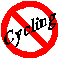Permutable subsets are concentrated within the array. Index values will not advance until all combinations are exhausted inside of the established range. After all unique combinations are exhausted, the upper index value is moved to the next level and the process repeats itself. All Examples presented here are concerned with the head or the tail of the target array. In this manner, concentrating exchanges within a prioritized list initially avoids irrational combinations and therefore prematurely solves NP-Complete problems incrementally (Promotes Scalable Permutation Solutions). In addition, solving problems incrementally clearly supports object threading in DNA genetic reconstruction projects based upon several known prime markers; this method is comparable to a polymerase chain reaction (or PCR) in small DNA samples...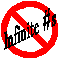Minimize counting and storage requirements. Yes, even today all computers still have limits and computing small factorial numbers will easily exceed them. (FACT: A common 32-bit integer cannot store 13 factorial!) Therefore, counting and memory requirements are less than or equal to the integer length of the target data structure (or the target array). Although every generation sequence is countable utilizing the Base-N-Odometer AND although all of the Examples provided here will handle very large arrays, you may not have the time to wait... NO VARIABLE DATA STACKS ALLOWED!
 `````` The Countdown QuickPerm Algorithm: let a[] represent an arbitrary list of objects to permute let N equal the length of a[] create an integer array p[] of size N+1 to control the iteration      initialize p to 0, p to 1, p to 2, ..., p[N] to N initialize index variable i to 1 while (i < N) do { decrement p[i] by 1 if i is odd, then let j = p[i] otherwise let j = 0 swap(a[j], a[i]) let i = 1 while (p[i] is equal to 0) do { let p[i] = i increment i by 1 } // end while (p[i] is equal to 0) } // end while (i < N) ``````
 `````` The Counting QuickPerm Algorithm: let a[] represent an arbitrary list of objects to permute let N equal the length of a[] create an integer array p[] of size N to control the iteration        initialize p to 0, p to 0, p to 0, ..., and p[N-1] to 0 initialize index variable i to 1 while (i < N) do { if (p[i] < i) then { if i is odd, then let j = p[i] otherwise let j = 0 swap(a[j], a[i]) increment p[i] by 1 let i = 1 (reset i to 1) } // end if else { // (p[i] equals i) let p[i] = 0 (reset p[i] to 0) increment i by 1 } // end else (p[i] equals i) } // end while (i < N) ``````

Before researching the actual C++ QuickPerm algorithms, study the "Base-N-Odometer" next.

[]

[] | [] | [] | [] | []
[] | []
[] | [] | []
[HOME]